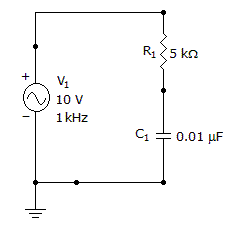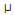# Electronics - Capacitors

### Exercise :: Capacitors - General Questions

16.

What is the phase shift between the voltage across the resistor and the voltage from the source?A. VR leads VS by 17° B. VR leads VS by 73° C. VR lags VS by 73° D. VR lags VS by 17°

Explanation:

No answer description available for this question. Let us discuss.

17.

A capacitor stores energy within a dielectric between the conducting plates in the form of:

 A. a magnetic field B. positive voltage C. negative voltage D. an electric field

Explanation:

No answer description available for this question. Let us discuss.

18.

As a capacitor is being charged, the voltage across its terminals will:

 A. increase B. decrease C. remain the same D. oppose the source

Explanation:

No answer description available for this question. Let us discuss.

19.

With a 500 kHz signal source, what would be the value of a capacitor yielding a capacitive reactance of 1 k?

 A. 318 pF B. 2 nF C. 3.18F D. 2 F

Explanation:

No answer description available for this question. Let us discuss.

20.

After a capacitor has charged for 1 tc, what percentage of current remains in the resistor?

 A. 63.2 B. 36.8 C. 13.5 D. 5